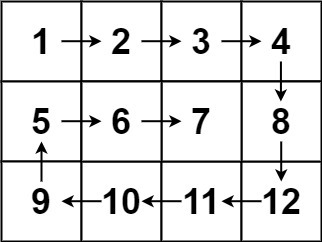# Spiral line

See the original problem on HackerRank.

A bunch of villains are waiting in line for being accepted on board of the Queen Anne’s Revenge, docked somewhere in Nassau, to become part of the crew. Every rascal is represented by a number corresponding to the “pieces of eight” they have stolen so far.

However, the pirates of the Queen Anne’s Revenge are as crazy as the captain and arrange that line in a spiral:Rascals that make it wrong will walk the plank!

We want to help them by writing an algorithm that prints the line from the outermost villain to the innermost. In other words, we want to print a matrix in clockwise order starting from the top left corner. For the example above, the expected output is:

1 2 3 4 8 12 11 10 9 5 6 7

Arrr!

### Input Format

• the first line contains $$N$$ (number of rows) and $$M$$ (number of columns).
• then, $$N$$ lines that contain $$M$$ numbers each.

### Constraints

• $$1 \leq N \leq 100$$
• $$1 \leq M \leq 100$$
• every number of the matrix is a 32-bit integer

### Output Format

The elements of the matrix printed in a clockwise spiral order, space-separated.

## Solutions

One solution consists in traversing the matrix left to right, then top to bottom, then right to left, then bottom to top, then repeat until we have done. Every time, the boundaries are updated. For example, when traversing from left to right, we are basically “cutting” the upper border of the matrix:

  1 2 3 4 5 6 7 8 9 10  1 2 3 4 12 13 14 5 11 16 15 6 10 9 8 7 ------> 1 2 3 4 12 13 14 5 11 16 15 6 10 9 8 7 

After visiting 1 2 3 4, the matrix turns into:

 1 2 3  12 13 14 5 11 16 15 6 10 9 8 7 

Then we visit the matrix from top to bottom:

 1 2 3  12 13 14 5 | 11 16 15 6 | 10 9 8 7 v 

And the matrix turns into:

 1 2 3  12 13 14 11 16 15 10 9 8 

And so on.

The core part of this problem is about updating indexes. If you don’t mess them up, you solve this problem easily.

Here is a C++ solution:

  1 2 3 4 5 6 7 8 9 10 11 12 13 14 15 16 17 18 19 20 21 22 23 24 25 26 27 28 29 30 31 32 33 34 35 36 37 38 39 40 41 42 43 44 45 46 47 48 49 50 51 52 53  int NumRows, NumColumns; cin >> NumRows >> NumColumns; const auto n = NumRows*NumColumns; vector matrix(n); copy_n(istream_iterator(cin), n, begin(matrix)); auto top = 0; auto bottom = NumRows-1; auto left = 0; auto right = NumColumns-1; int direction = 0; const auto updateOutput = [=](int i, int j) { cout << matrix[i*NumColumns + j] << " "; }; while (top <= bottom && left <= right) { switch (direction) { case 0: for (auto i=left; i<=right; ++i) { updateOutput(top, i); } direction = 1; ++top; break; case 1: for (auto i=top; i<=bottom; ++i) { updateOutput(i, right); } direction = 2; --right; break; case 2: for (auto i=right; i>=left; --i) { updateOutput(bottom, i); } direction = 3; --bottom; break; case 3: for (auto i=bottom; i>=top; --i) { updateOutput(i, left); } direction = 0; ++left; break; } } 

From here, we can even make the solution more concise and generic by introducing a sort of direction vector that contains the offsets of all the directions to take:

 1  [0, 1], [1, 0], [0, -1], [-1, 0] 

For example, [0, 1] means left to right: 0 represents the steps on the row axis, 1 on the column axis.

Here is the complete solution in C++:

  1 2 3 4 5 6 7 8 9 10 11 12 13 14 15 16 17 18 19 20 21  int NumRows, NumColumns; cin >> NumRows >> NumColumns; const auto n = NumRows*NumColumns; vector matrix(n); copy_n(istream_iterator(cin), n, begin(matrix)); const vector> offsets{{0, 1}, {1, 0}, {0, -1}, {-1, 0}}; vector remainingSteps{NumColumns, NumRows-1}; auto currentDirection = 0; auto stepRow = 0, stepCol = -1; while (remainingSteps[currentDirection%2]) { for (int i = 0; i < remainingSteps[currentDirection%2]; ++i) { stepRow += offsets[currentDirection]; stepCol += offsets[currentDirection]; cout << matrix[stepRow*NumColumns + stepCol] << " "; } remainingSteps[currentDirection%2]--; currentDirection = (currentDirection + 1) % 4; } 

In addition to being more succinct, this solution is more generic and allows us to change direction easily. For instance, the only things to change in order to visit the matrix in counterclockwise order are:

 1  const vector > offsets{{1, 0}, {0, 1}, {-1, 0}, {0, -1}}; 

and

 1  auto x = -1, y = 0; 
We've worked on this challenge in these gyms: modena